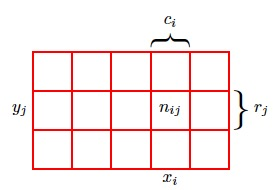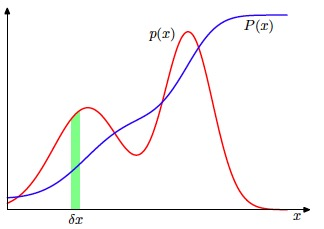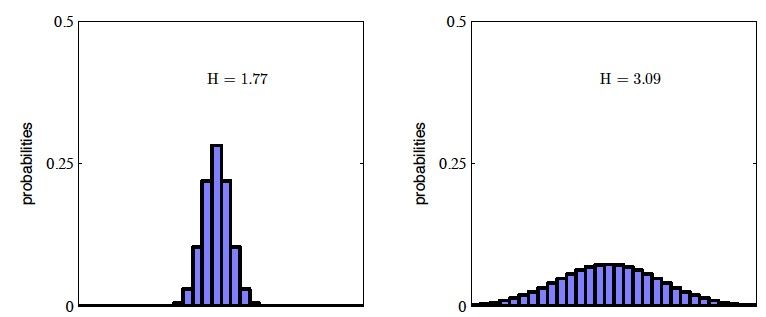2017-03-17

# 1.1 曲线拟合

$y(x,\mathbb{w}) = w_0 + w_1x + w_2x^2 +... + w_Mx^M = \sum_{j=0}^{M}w_jx^j$

• 过拟合 当我们达到更⾼阶的多项式（M = 9），我们得到了对于训练数据的⼀个完美的拟 合。事实上，多项式函数精确地通过了每⼀个数据点，$E(w) = 0$。然⽽，拟合的曲线剧烈震 荡，就表达函数$sin(2\pi x)$⽽⾔表现很差。这种⾏为叫做过拟合（over-fitting）。

# 1.2 概率论图1.9

## 加和规则和乘积规则图1.10

$p(X = x_i, Y = y_j) = \frac{n_{ij}}{N}$

$p(X=x_i) = \frac{c_i}{N}$

$p(X=x_i) = \sum_{j}^{L}p(X=x_i,Y=y_j)$

$p(X = x_i)$有时被称为边缘概率（marginal probability），因为它通过把其他变量（本例中的Y ）加总到边缘或者加和得到。(类似excel表格总计一般是统计到表格的边缘). 如果我们只考虑那些$X =x_i$的实例，那么这些实例中$Y =y_j$的实例所占的⽐例被写成$p(Y = y_j|X = x_i)$，被称为给定$X = x_i$的$Y = y_j$的条件概率（conditional probability）。它的 计算⽅式为：计算落在单元格i, j的点的数量与列i的点的数量的⽐值，即

$p(Y = y_j|X = x_i) = \frac{n_{ij}}{c_i}$

$p(X=x_i,Y=y_j) =\frac{n_{ij}}{N}=\frac{n_{ij}}{c_i}.\frac{c_i}{N} = p(Y = y_j|X = x_i)p(X=x_i)$

$p(X)=\sum_Y p(X,Y) p(X,Y) = p(Y|X)p(X)$
 其中p(X,Y )是联合概率，可以表述为“X且Y 的概率”。类似地，p(Y X)是条件概率，可以表述为“给定X的条件下Y 的概率”，p(X)是边缘概率，可以简单地表述为“X的概率”。这两个简单的规则组成了我们在全书中使⽤的全部概率推导的基础。

$p(Y|X) = \frac{P(X,Y)}{P(X)}$

$p(Y | X) = \frac {p(X | Y )p(Y )}{p(X)}$

## 1.2.1 连续变量和概率密度

$p(x \in (a, b)) = \int_{a}^{b}p(x) dx$

$p(x)>0 \int_{-\infty}^{\infty}p(x)dx=1$图1.12

$p(x)>0 \int_{-\infty}^{\infty}p(x)dx=1$

$p(x) = \int p(x)dx p(x,y) = p(y|x)p(x)$

## 1.2.2 期望和协⽅差

$E(f) = \sum_x p(x)f(x)$

$E(f) = \int_x p(x)f(x)dx$

$E(f) = \frac{1}{N}\sum_{n=1}^N f(x_n)$

f(x)的⽅差（variance）被定义为

$var[f] = E[(f(x)-E[f(X))^2] var[f] = E[f(x)^2-E[f(X)]^2 var[x] = E(x^2)-E(x)^2$

$cov[x, y] = E_{x,y}[\{x-E[x]\}\{y^T - E[y^T]\}] = E_{x,y}[xy^T]-E[x]E[y^T]$

$cov[x, y] = E_{x,y}[\{x-E[x]\}\{y^T - E[y^T]\}] = E_{x,y}[xy^T]-E[x]E[y^T]$

$cov[x] \equiv cov[x, x]$

## 1.2.3 贝叶斯概率

$p(w | D) = \frac{p(D | w)p(w)} {p(D)}$

$posterior \varpropto likelihood \times prior$
• 独立同分布 独⽴地从相同的数据点中抽取的数据点被称为独⽴同分布（independent and identically distributed），通常缩写成i.i.d.

• 最大似然估计 两个独立事件的联合概率等于独立事件的概率的乘积. 那么:

$p(x|\mu,\sigma)=\prod_{n=1}^{N}\mathcal N(x_n|u,\sigma^2)$

# 1.5 决策论

$p(C_k|x) = \frac{p(x|C_k)p(C_k)}{p(x)}$

 把分类问题划分成了两个阶段：推断（inference）阶段和决策（decision）阶段。在推断阶段，我们使⽤训练数据学习$p(C_k x)$的模型。在接下来的决策阶段，我们使⽤这些后验概率来进⾏最优的分类。另⼀种可能的⽅法是，同时解决两个问题，即简单地学习⼀个函数，将输⼊x直接映射为决策。这样的函数被称为判别函数（discriminant function)

1.  对于每个类别$C_k$，独⽴地确定类条件密度$p(x C_k)$。这是⼀个推断问题。然后，推断先验类概率$p(C_k)$。之后，使⽤贝叶斯定理
$p(C_k|x) = \frac{p(x|C_k)p(C_k)}{p(x)} p(x)=\sum_k p(x|C_k)p(C_k)$

 我们可以直接对联合概率分布$p(x C_k)$建模，然后归⼀化，得到后验概率。得到后验概率之后，我们可以使⽤决策论来确定每个新的输⼊x的类别。显式地或者隐式地对输⼊以及输出进⾏建模的⽅法被称为⽣成式模型（generative model），因为通过取样，可以⽤来⼈⼯⽣成出输⼊空间的数据点。
1.  ⾸先解决确定后验类密度$p(C_k x)$这⼀推断问题，接下来使⽤决策论来对新的输⼊x进⾏分类。这种直接对后验概率建模的⽅法被称为判别式模型（discriminative models）。
2. 找到⼀个函数f(x)，被称为判别函数。这个函数把每个输⼊x直接映射为类别标签。例 如，在⼆分类问题中，f()可能是⼀个⼆元的数值，f = 0表⽰类别$C_1$，f = 1表⽰类别$C_2$。这种情况下，概率不起作⽤。 

# 1.6 信息论

我们考虑⼀个离散的随机变量x。当我们观察到这个变量的⼀个具体值的时候，我们接收到了多少信息呢？信息量可以被看成在学习x的值的时候的“惊讶程度”。 我们对于信息内容的度量将依赖于概率分布p(x)，因此我们想要寻找⼀个函数h(x)，它 是概率p(x)的单调递增函数，表达了信息的内容。h()的形式可以这样寻找：如果我们有两 个不相关的事件x和y，那么我们观察到两个事件同时发⽣时获得的信息应该等于观察到事 件各⾃发⽣时获得的信息之和，即$h(x, y) = h(x) + h(y)$。两个不相关事件是统计独⽴的，因 此$p(x, y) = p(x)p(y)$。根据这两个关系，很容易看出$h(x)$⼀定与p(x)的对数有关。因此，我们有

$h(x) = log_2 p(x)$

$H[x] = - \sum_x p(x) log_2 p(x)$

## 示例

$H[x] = -8 \times \frac{1}{8} \times log_2 {\frac{1}{8}} = 3 bit$

$H[x] = 2bit$图1.30 两个概率分布在30个箱⼦上的直⽅图，表明熵值越⼤，H越宽。最⼤的熵值产⽣于均匀分布，此 时的熵值为H=-ln(1/30)=3.40

 假设我们有⼀个联合概率分布p(x,y)。我们从这个概率分布中抽取了⼀对x和y。如果x的值已知，那么需要确定对应的y值所需的附加的信息就是􀀀lnp(y x)。因此，⽤来确定y值的平均附加信息可以写成
$H[y | x] =\iint p(y, x) ln p(y , x) dy dx$